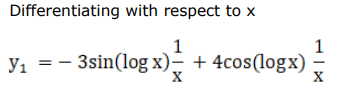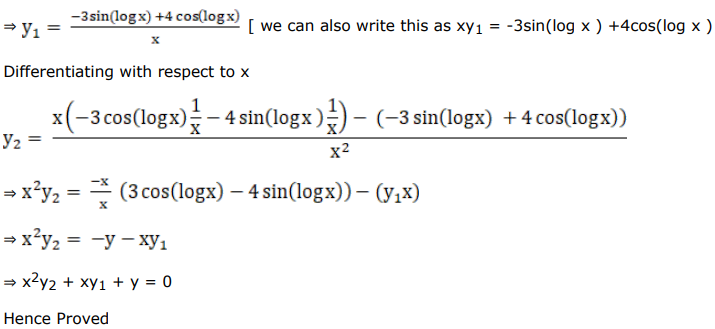# Solve this followingQuestion:

If $y=3 \cos (\log x)+4 \sin (\log x)$, prove that $x^{2} y_{2}+x y_{1}+y=0$

Solution: